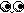## The Code

Folder:
Most Recent

W  W  W  W

E   E  E   E

W   W

T  B  S  T   E  E

A  R  E  H

S  E  E  I   A  A

T  A     N  R  R

E  T  W  K  E   E

H  I

W  E  T  W N   M

I       H  I  O E

T  W     T  T   R

H  I  N  H     E

T  O      H   L

N  H      N  U   Y

O      E  O  M

N  Y       A   M

T  O  E  M  N   A

O      S  I  S    C

U  L      N  .   H

N  U      D  .  I

G   N           N

E  G            E

S            S

.View foxgloves's Full Portfolio